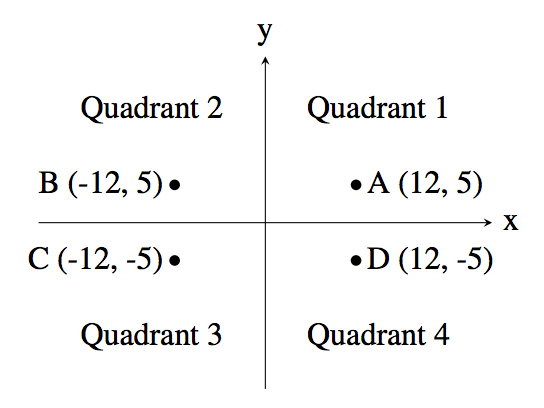A common problem in mathematics is to determine which quadrant a given point lies in. There are four quadrants, numbered from 1 to 4, as shown in the diagram below:For example, the point A, which is at coordinates (12, 5) lies in quadrant 1 since both its x and y values are positive, and point B lies in quadrant 2 since its x value is negative and its y value is positive.

Your job is to take a point and determine the quadrant it is in. You can assume that neither of the two coordinates will be 0.

### Input Format

The first line of input contains the integer x (−1000 ≤ x < 1000; x ≠ 0). The second line of input contains the integer y (−1000 ≤ y ≤ 1000; y ≠ 0).

### Output Format

Output the quadrant number (1, 2, 3 or 4) for the point (x, y).

```12
5
```

```1
```

```9
-13
```

### Sample Output 2

```4
```

Point Value: 3
Time Limit: 1.00s
Memory Limit: 16M